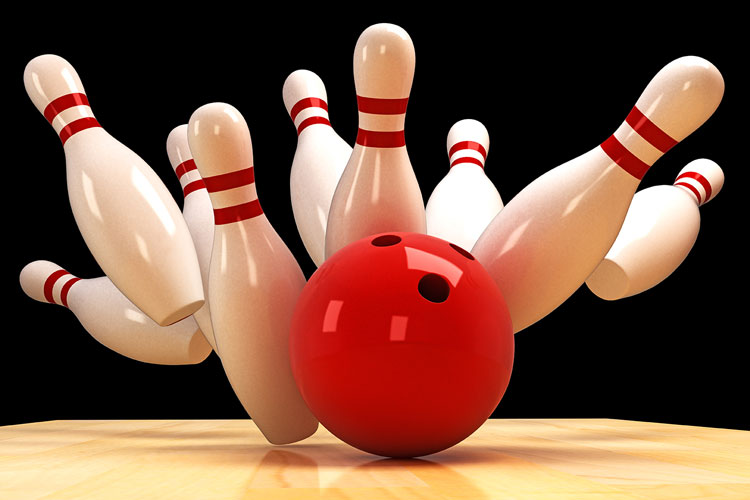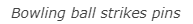STEM Labs
Momentum Alley Teacher

Teacher Portal# The Science of Momentum

Momentum can be thought of as the amount of motion that an object has. Momentum is determined by the object's mass (how much is moving) and its velocity (how fast it is moving). In physics, momentum is represented by "p" so the equation for determining momentum is p = mv (momentum equals mass times velocity). A heavier object, like a bowling ball, traveling at the same speed as a lighter object, like a kickball, would have greater momentum according to the laws of physics.

For this STEM Lab, you need to understand momentum and how it affects collisions because later, you will compete in a bowling game called the Strike Challenge. You will program the Speedbot to run into a ball in order to knock down pins and earn the highest score. Let's think about what happens when two objects collide. They transfer kinetic energy, yes. But, the momentum of each of the two colliding objects before the collision can predict how the two objects will react after the collision. We will return to this idea later.Motivate Discussion

Reasoning about momentum within a discussion is a good way to guide your students' efforts to conceptualize it. The focus of this STEM Lab will be on the momentum of the robot as it collides with a ball.

The following questions can be reasoned about in metric units as written, or in imperial miles, pounds, and feet per second. The reasoning should be the same without the need for conversions.

Q: Which of these has more momentum: a sphere rolling at 8 km per hour or the same sphere rolling at 10 km per hour?
A: The sphere rolling at 10 km per hour has more momentum because it's moving faster.

Q: Which of these has more momentum: an average sized car driving at 90 km per hour or a bus driving at 90 km per hour?
A: The bus driving at 90 km per hour has more momentum because it has more mass.

Q: Which of these has more momentum: a bicycle being pedaled up a hill or the same bicycle being pedaled down a hill?
A: The bicycle being pedaled down the hill has more momentum because the downward ramp of the hill and the pedaling are both accelerating its speed - unless a braking system is also being applied.

Q: Which would you rather have collide with you: a 1000 kg truck moving at 1 meter per minute or a 1 kg melon moving at 1000 meters per second?
A: The truck might knock you over but it is not moving fast enough to seriously injure you whereas the melon moving at 1000 m/s could kill you. This highlights that the amount of momentum is related to the amount of energy transferred from an object during a collision. The mass of an object alone isn't enough to determine how much destructive energy it supplies during a collision. We also need to consider how fast it is moving.